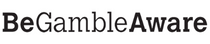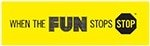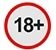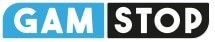# Betting Odds Explained. Guide – Betting School – helpbetA first thing associated with sports betting is the betting odds. No punter can place bets without understanding what all those decimal numbers or various mathematical symbols mean. So, it goes without saying that before placing your first bet (while you find yourself in the  you should learn all about betting odds.

### Betting Odds Explained. Importance of Understanding Betting Odds

Betting odds are crucial when it comes to generating profit in the long run. Betting odds are much more than weird numbers as they help punters calculate both probability and possible winnings.

The higher the probability of something happening, the higher your chances of winning your bet are. However, better odds in terms of probability result in lower payouts. Plus, different online bookmakers offer different odds i.e. placing the identical bet at two separate betting sites may result in different returns.

Thus, knowing how football betting odds work and what they represent is a must. Without understanding the odds, you will probably place bets based on personal opinion, which often may result in generating losses.

### Betting Odds Types and How to Convert Them

First thing sports bettors should know is that there are three basic types of odds; these are: decimal, fractional and moneyline or American odds. All of them are represented in a different way and it’s smart to understand how each one of them works.

### Decimal Odds

Decimal odds are in the form of 1.25, 2.95 and so on. Converting decimal betting odds to probabilities is done via a simple calculation that involves dividing the figure one by the decimal odds and then multiplying the result with 100. For example, if the odds of Cristiano Ronaldo scoring a goal are 1.45, you should do the following:

1st step: 1 / 1.45 = 0.689

2nd step: 0.689 * 100 = 68.9%

Therefore, the probability of Cristiano Ronaldo scoring a goal is 68.9%.

###Fractional Odds Explained

Let us now focus on fractional or UK Betting Odds; You can see these as 5/2, 1/1 etc. Calculating the probability of a bet using fractional odds seems a bit more complicated. The formula for this would be dividing the denominator by the result from the sum of the denominator and the numerator. Finally, the result is multiplied by 100 in order to get the wanted percentage. To make this easier to understand, let us provide you with an example.

For instance, we want to place a bet that Cristiano Ronaldo won’t score a goal with fractional odds 5/2; here, the denominator is the figure 2, while the numerator is the figure 5. Using the previously stated formula, what we need to do is the following:

1st step: 2 / (2 + 5)

2nd step: divide 2 by 7 and get the result of 0.2857

3rd step: 0.285 is multiplied by 100 to give the final result of 28.57% which is the potential winnings possibility.

### Moneyline Odds (American Odds)

Finally, it’s time to explain the moneyline odds or also known as American odds. Just like their name suggests, these are mostly used in the United States, especially when it comes to basketball and baseball betting.

Moneyline bets are represented with either positive or negative figures i.e. you may see a figure with either a “-“ or a “+” in front of it. For example, let’s say that Golden State Warriors are favourites in a basketball game and their monelyline betting odds are -120. On the other hand, Los Angeles Lakers are expected to lose the game and their odds are +180. The question here is how to calculate the probability of either bet happening in percentage.

Theoretically speaking, the formula for that would be to neutralize the negative moneyline odds and then divide them by the sum between neutralized moneyline odds and the number 100. Finally, multiply by 100 to get the percentage. Sounds confusing? Well, let’s show it in practice.

1st step: turn -120 into 120

2nd step: sum neutralized 120 and 100 to get 220

3rd step: divide the first 120 by 220 (the result of step 2)

4th step: multiply the result from step 3 i.e. 0.545 by 100 to get the final result of 54.5%

### Calculating Winnings

Not only betting odds help punters calculate the probability of something happening, but they help to determine the possible winnings too. Namely, regardless of the type of odds, the figures used to represent them reveal a lot.

Here’s one example. Let’s say the odds for a bet you want to place are 8/1; this means that for every £1 you place you will win £8 if the bet is winning. On the other hand, if the odds are 1/8, you can expect to win modest £1 for every £8 you bet.

Now, let’s see a few examples for calculating winnings with decimal odds. These may seem a bit more confusing though. For instance, if the odds are 8.0, you should multiply the odds with the bet amount and then subtract the bet amount from the result i.e. use this formula (8.0 * £10 bet) – £10 bet; the final result i.e. the winnings are £70. Same goes for betting £10 with 3.5 odds; your winnings in this scenario would be £25.

Finally, let’s analyze the moneyline odds. If you bet on the negative odds i.e. -150, you get £100 for every £150 wagered. On the other hand, betting on the positive odds i.e. +190 means that you get £190 for every £100 wagered.

### 100% Welcome Bonus up to £250 + £10 Casino Bonus